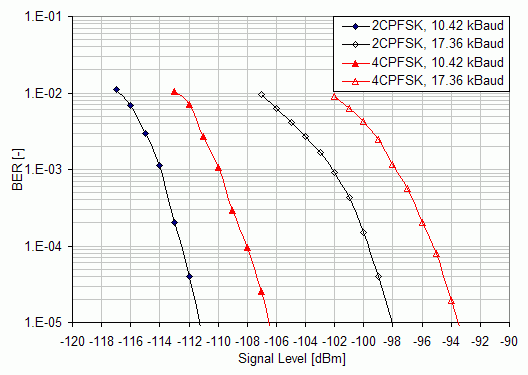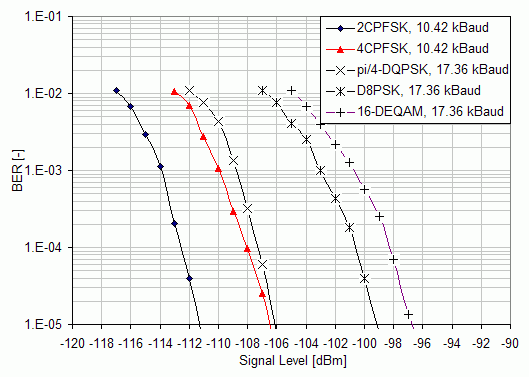Print version

The most important parameters which describe the quality of narrowband radio receiver are maximum usable (data) sensitivity, co-channel rejection, adjacent channel selectivity, desensitization and intermodulation response rejection. Besides the maximum usable sensitivity, all other receiver parameters can be classified as the measures of the receiver degradation parameters used to analyze the degradation of its performance due to the presence of unwanted (interfering) signals. Although there is a strong relation between all of these parameters, in this paper the attention is given only to the first of them, to the maximum usable sensitivity in particular.

According to , the maximum usable data sensitivity is the minimum level of the signal (emf) at the receiver input, produced by a carrier at the nominal frequency of the receiver, modulated with a normal test signal, which will, without interference, produce, after demodulation, a data signal with a specified bit-error-ratio (BER) of 10-2 or a specified successful message ratio (SMR) of 80%.

 The maximum usable sensitivity shall not exceed an electromotive force of 3.0 dBμV under normal test conditions.

Assigning this value as S, one can also express what signal-to-noise ratio (SNR) can be expected in relation to noise figure (NF) and transformed to the receiver input

`                        SNR = S -(10.log(kT)+10.log(BN)+NF)  [dB].               (2.1)`

In (2.1), k is the Boltzmann’s constant, T is the absolute temperature in Kelvin and BN is the receiver noise bandwidth of e.g. 25 kHz.

## 2.1. Maximum usable data sensitivity

In this section, the results of maximum usable data sensitivity measurement (Figure 3.4) for the complete narrowband radio transceiver are presented. All the results are given for 25 kHz channel separation.

Firstly, let us focus on operational modes with exponential modulations, Figure 3.4. It can be seen that the emf sensitivity limit of +3 dBμV (-110 dBm @ 50 Ω) is fulfilled with margin for both modulations (2CPFSK, 4CPFSK) when running at the symbol rate of 10.4 kBaud. When higher symbol rates are selected, these modulations loss their power efficiency rapidly and for the selected symbol rate of 17.3 kBaud, the sensitivities lower down to the values of −107 dBm @ BER=10-2 and −102 dBm @ BER=10-2 for 2CPFSK and 4CPFSK respectively. This discrepancy is caused mainly due to the fact that there is a significantly lower frequency deviation used at the higher symbol rates. The decrease in power efficiency with increasing spectrum efficiency is not linear as for the typical linear modulations. Although possible, this example documents that the increase in spectrum efficiency of exponential modulation techniques cannot be considered for efficient use of assigned bandwidth.Fig. 2.1: Maximum usable sensitivity measurement results for different settings of exponential modulations.

The second set of measurement results, presented in Figure 3.5, documents the power efficiency analysis of operational modes based on the linear modulation techniques. It can be seen that when using the linear π/4-DQPSK, the radio receiver can still reach the data sensitivity limit even for 17.3 kBaud with a 2 dB margin. Even from this comparison it is evident that the π/4-DQPSK mode of operation outperforms the 4-CPFSK at higher spectrum efficiencies. Further increase in spectrum efficiency can be reached by higher order constellations such as D8PSK and 16DEQAM and the radio receiver can still maintain practically applicable sensitivities of −107 dBm @ BER=10-2 and −105 dBm @ BER=10-2 respectively.Fig. 2.2: Maximum usable sensitivity measurement results. Channel separation 25 kHz.

## 2.2. Efficient use of narrowband radio channel

As it has been written in the Section 1, the radio transceiver in exponential modulation mode can make use of higher transmitter power. In order to take this fact into account the system gain (SG) or the maximum allowed path loss (2.2)

`                        SG [dB] = Pout- S ,                                       (2.2)`

is usually calculated for the wireless communication systems. Here the Pout is the available transmitter power expressed in dBm and S is the measured value of radio receiver sensitivity, also in dBm. It expresses the referential value of the link budget, assuming 0 dBi of antennas gain and together with the spectrum efficiency given by (2.3) it expresses how effectively the radio device uses its assigned bandwidth

```                                        Rb
η [bit/s/Hz] = —— .                                      (2.3)
B```

In (2.3), the Rb is the raw bit rate given in [bits/s] and B is the frequency bandwidth assigned to the radio system, 25 kHz in particular.

All these performance characteristics are collectively given in Table 3.2. It can be seen that even with the lower available transmitter power, the radio transceiver can reach wider system gain at higher spectrum efficiencies while running in linear as oppose to the exponential modulation mode. On the other hand, if the long distance coverage is of the primary application concern, even the 2CPFSK modulation having spectrum efficiency of 0.4 bit/s/Hz, but the system gain of impressive, 157 dB, can be a reasonable option.

Tab. 2.1: Overall performance characteristics of the narrowband radio transceiver for selected modes of operation.

Modulation FormatModul. Param.Symbol RateRaw Bit RateSpectrum EfficiencyData
Sensitivity
@ BER 10-2
Available Output PowerSystem Gain
[-][-][kBaud][kbits/s][bit/s/Hz][dBm][dBm][dB]
2CPFSKh=0.6, α=0.2810.4210.420.42-11740157
h=0.2, α=0.2817.3617.360.69-10740147
4CPFSKh=0.3, α=0.2810.4220.830.83-11340153
h=0.1, α=0.2817.3634.721.39-10240142
π/4-DQPSKα=0.417.3634.721.39-11235147
D8PSKα=0.417.3652.082.08-10735142
16-DEQAMα=0.417.3669.442.78-10533138
Measurement uncertainty ±2 dB